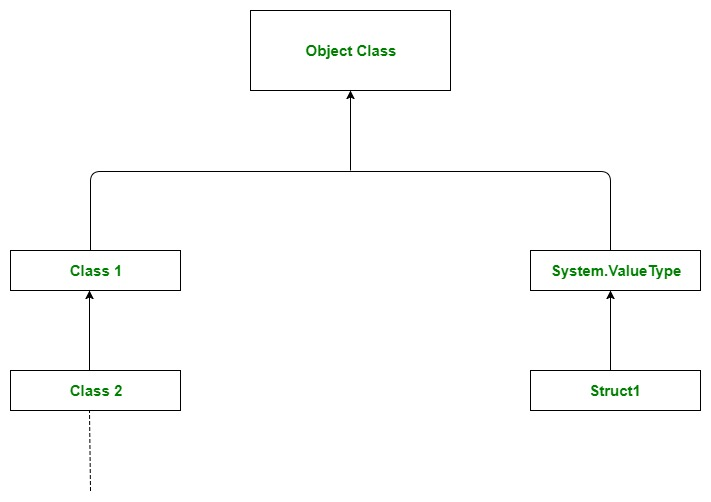# Vector clone() Method in Java with Examples

The clone() method of the Vector class is used to return a shallow copy of this vector provided by the Object class. It just creates a copy of the vector. The copy will have a reference to a clone of the internal data array but not a reference to the original internal data array.

Syntax:

`Vector.clone()`

Parameters: The method does not take any parameters.

Return Value: The method returns an Object which is just the copy of the vector.

Exception Thrown: CloneNotSupportedException: This exception is thrown if the object’s class does not support the Cloneable interface.

Example 1:

## Java

 `// Java Program to Illustrate clone() method of Vector class`   `// Importing required classes` `import` `java.util.*;`   `// Main class` `// VectorDemo` `public` `class` `GFG {`   `    ``// Main driver method` `    ``public` `static` `void` `main(String args[])` `    ``{`   `        ``// Creating an empty Vector by creating object` `        ``// of vector class of string type` `        ``Vector vec_tor = ``new` `Vector();`   `        ``// Adding custom input elements into the vector` `        ``// using add() method` `        ``vec_tor.add(``"Welcome"``);` `        ``vec_tor.add(``"To"``);` `        ``vec_tor.add(``"Geeks"``);` `        ``vec_tor.add(``"For"``);` `        ``vec_tor.add(``"Geeks"``);`   `        ``// Print and display all vector elements` `        ``System.out.println(``"Vector: "` `+ vec_tor);`   `        ``// Creating another vector to copy by` `        ``// creating an object of Object class` `        ``Object copy_vector = vec_tor.clone();`   `        ``// Print and display the cloned vector elements` `        ``System.out.println(``"The cloned vector is: "` `                           ``+ copy_vector);` `    ``}` `}`

Output

```Vector: [Welcome, To, Geeks, For, Geeks]
The cloned vector is: [Welcome, To, Geeks, For, Geeks]```

Example 2:

## Java

 `// Java Program to Illustrate clone() method of Vector class`   `// Importing required classes` `import` `java.util.*;`   `// Main class` `// VectorDemo` `public` `class` `VectorDemo {` `  `  `    ``// Main driver method ` `    ``public` `static` `void` `main(String args[])` `    ``{` `        ``// Creating an empty Vector by creating` `       ``// object of Vector class of Integer type ` `        ``Vector vec_tor = ``new` `Vector();`   `        ``// Add custom input elements for  ` `        ``// using add() method` `        ``vec_tor.add(``10``);` `        ``vec_tor.add(``15``);` `        ``vec_tor.add(``30``);` `        ``vec_tor.add(``20``);` `        ``vec_tor.add(``5``);`   `        ``// Print and display the vector elements ` `        ``System.out.println(``"Vector: "` `+ vec_tor);`   `        ``// Creating another vector to copy by` `        ``// creating object of Object class ` `        ``Object copy_vector = (Vector)vec_tor.clone();`   `        ``// Print and display elements of cloned vector ` `        ``System.out.println(``"The cloned vector is: "` `+ copy_vector);` `    ``}` `}`

Output

```Vector: [10, 15, 30, 20, 5]
The cloned vector is: [10, 15, 30, 20, 5]```Note: In the above examples we have cloned vectors by creating objects of Object class itself as it does provide clone() method. So if object classes are not supporting any cloneable interface then it will not allow to clone and copy vector elements so does it will throw CloneNotSupportedException.

Whether you're preparing for your first job interview or aiming to upskill in this ever-evolving tech landscape, GeeksforGeeks Courses are your key to success. We provide top-quality content at affordable prices, all geared towards accelerating your growth in a time-bound manner. Join the millions we've already empowered, and we're here to do the same for you. Don't miss out - check it out now!